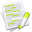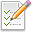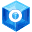#wx.Point¶

A wx.Point is a useful data structure for graphics operations.

It contains integer x and y members. See wx.RealPoint for a floating point version.

Note that the width and height stored inside a wx.Point object may be negative and that wx.Point functions do not perform any check against negative values (this is used to e.g. store the special -1 value in wx.DefaultPosition instance).

wx.RealPoint

##Class Hierarchy¶Inheritance diagram for class Point:

##Methods Summary¶

 __init__ Constructs a point. Get Return the x and y properties as a tuple. GetIM Returns an immutable representation of the wx.Point object, based on namedtuple. IsFullySpecified Returns True if neither of the point components is equal to DefaultCoord. SetDefaults Combine this object with another one replacing the uninitialized values. __eq__ __getitem__ __len__ __ne__ __reduce__ __repr__ __setitem__ __str__ __iadd__ __isub__

##Properties Summary¶

 IM x A public C++ attribute of type int. x member. y A public C++ attribute of type int. y member.

##Class API¶

class wx.Point(object)

Possible constructors:

Point()

Point(x, y)

Point(pt)


A Point is a useful data structure for graphics operations.

### Methods¶

__init__(self, *args, **kw)Overloaded Implementations:

__init__ (self)

Constructs a point.

Initializes the internal x and y coordinates to zero.

__init__ (self, x, y)

Initializes the point object with the given x and y coordinates.

Parameters
• x (int) –

• y (int) –

__init__ (self, pt)

Converts the given wx.RealPoint (with floating point coordinates) to a wx.Point instance.

Notice that this truncates the floating point values of pt components, if you want to round them instead you need to do it manually, e.g.

rp = wx.RealPoint(10.3, 20.8)
p = wx.Point(int(round(rp.x)), int(round(rp.y)))

Parameters

pt (wx.RealPoint) –

Get(self)

Return the x and y properties as a tuple.

Return type

tuple

Returns

( x, y )

GetIM(self)

Returns an immutable representation of the wx.Point object, based on namedtuple.

This new object is hashable and can be used as a dictionary key, be added to sets, etc. It can be converted back into a real wx.Point with a simple statement like this: obj = wx.Point(imObj).

IsFullySpecified(self)

Returns True if neither of the point components is equal to DefaultCoord.

This method is typically used before calling SetDefaults .

Return type

bool

New in version 2.9.2.

SetDefaults(self, pt)

Combine this object with another one replacing the uninitialized values.

It is typically used like this:

if not pos.IsFullySpecified():

pos.SetDefaults(GetDefaultPosition())

Parameters

pt (wx.Point) –

New in version 2.9.2.

__eq__(self, other)
Return type

bool

__getitem__(self, idx)

__len__(self)

__ne__(self, other)
Return type

bool

__reduce__(self)

__repr__(self)

__setitem__(self, idx, val)

__str__(self)

__iadd__(self, *args, **kw)Overloaded Implementations:

Parameters

sz (wx.Size) –

Parameters

pt (wx.Point) –

__isub__(self, *args, **kw)Overloaded Implementations:

__isub__ (self)

Parameters

sz (wx.Size) –

__isub__ (self)

Parameters

pt (wx.Point) –

### Properties¶

IM

x

A public C++ attribute of type int. x member.

y

A public C++ attribute of type int. y member.